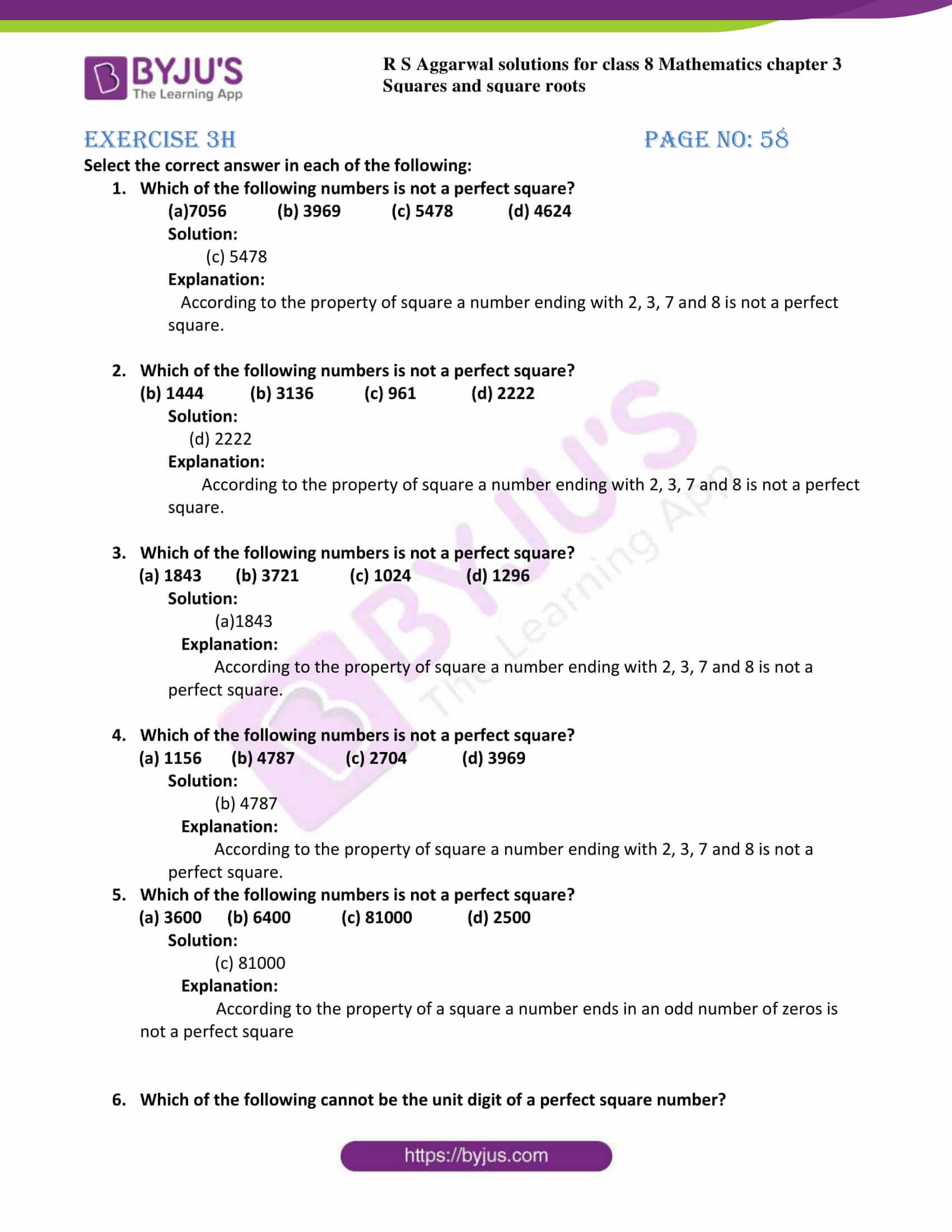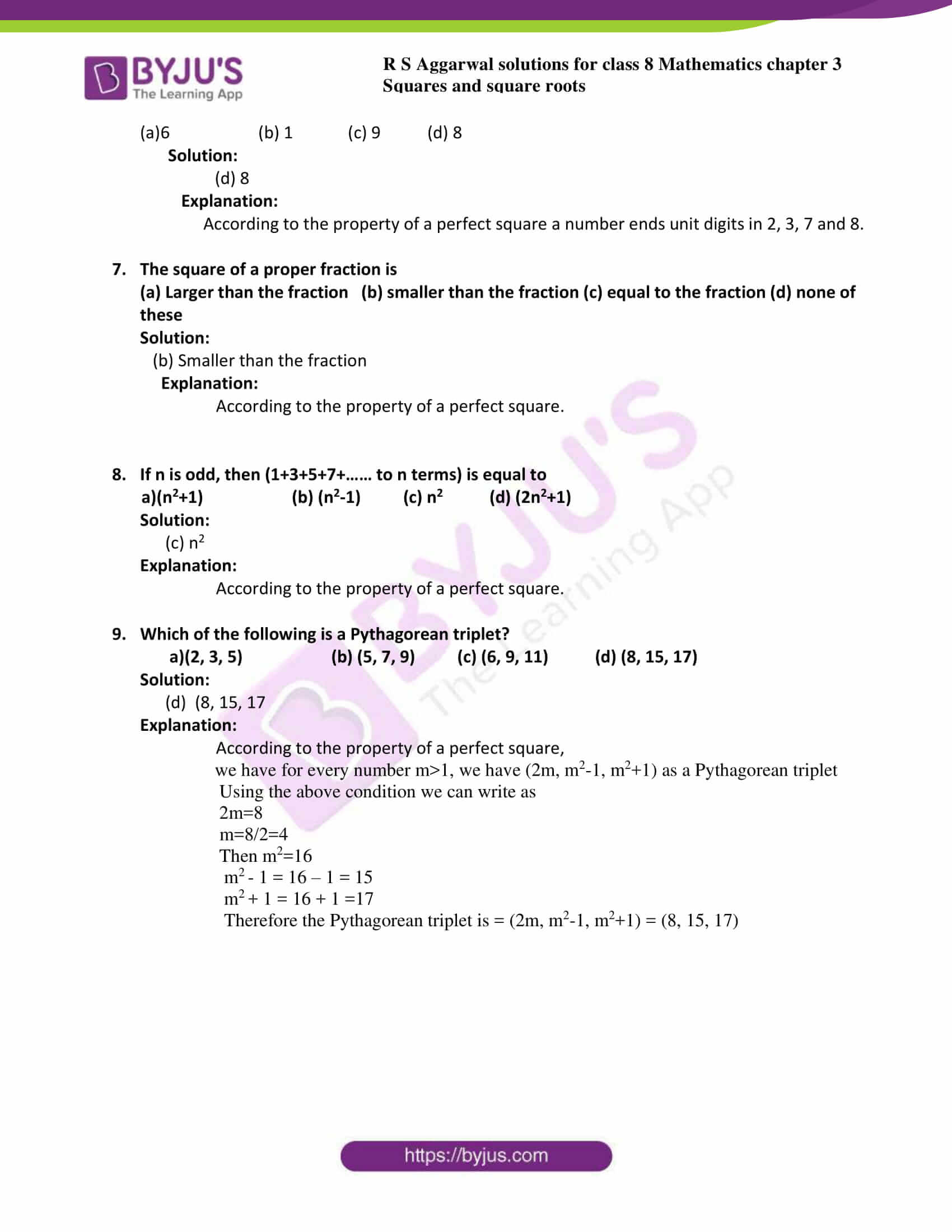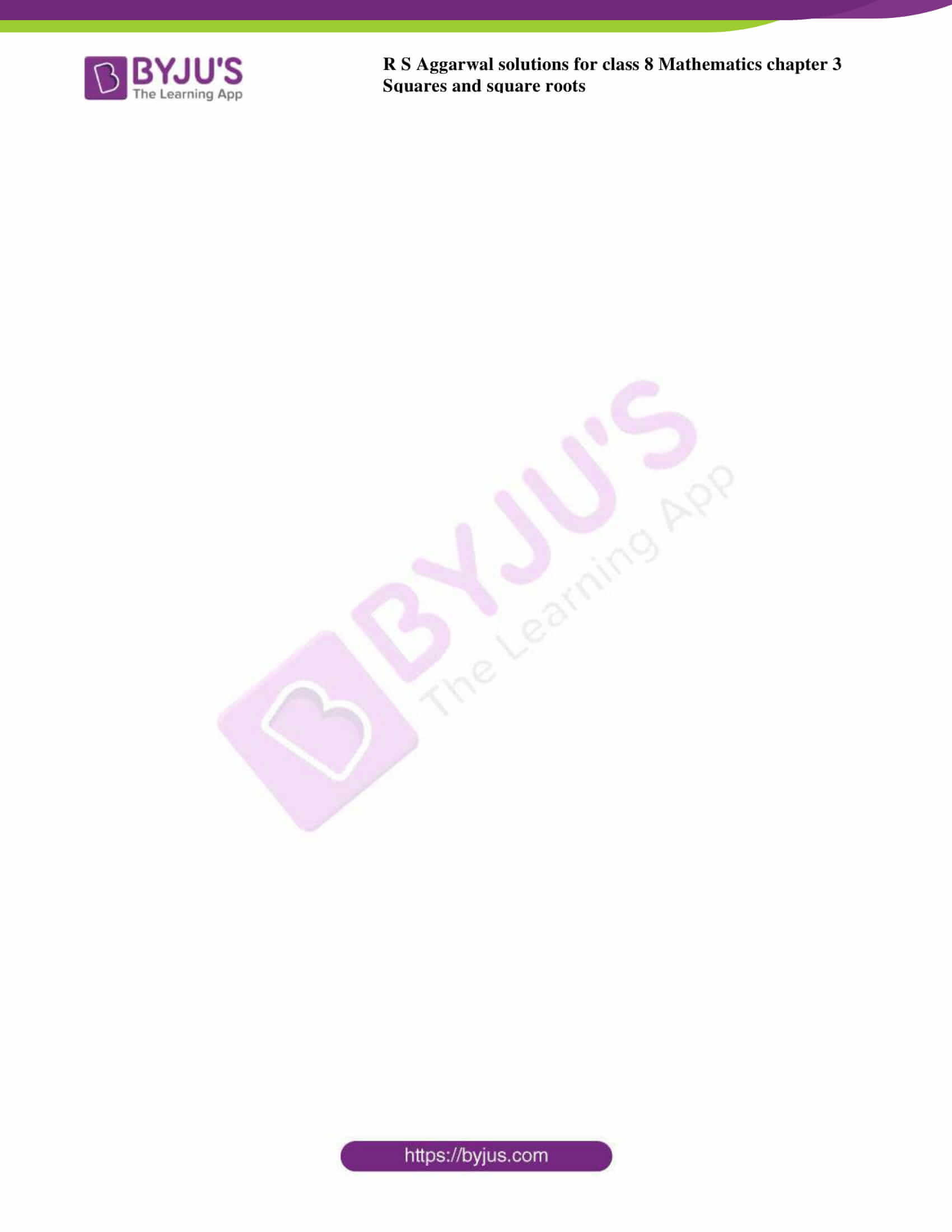# RS Aggarwal Solutions for Class 8 Chapter 3 - Square and Square Roots Exercise 3H

Students can refer and download the RS Aggarwal Solutions for the Exercise 3H of Class 8 Maths Chapter 3, Squares and Square roots from the link available here. Our experts have solved the RS Aggarwal Solutions to ensure that the students are thorough with their concepts by practising the solutions.

In exercise 3H we shall study the concepts covered across the whole chapter. This includes the definition of squares, perfect squares or square numbers, properties of perfect squares, product of two consecutive odd or consecutive even numbers, methods for squaring a number, square roots, methods to find square root of a perfect square, square roots of numbers in decimal form, square roots of fraction. By practising the RS Aggarwal Solutions for class 8, students will be able to grasp the concepts perfectly.

## Download PDF of RS Aggarwal Solutions for Class 8 Chapter 3 – Squares and Square Roots – Exercise 3H### Access answers to Maths RS Aggarwal Solutions for Class 8 Chapter 3 – Squares and Square Roots Exercise 3H

Select the correct answer in each of the following:

1. Which of the following numbers is not a perfect square?

(a)7056 (b) 3969 (c) 5478 (d) 4624

Solution:

(c) 5478

Explanation:

According to the property of square a number ending with 2, 3, 7 and 8 is not a perfect square.

2. Which of the following numbers is not a perfect square?

(b) 1444 (b) 3136 (c) 961 (d) 2222

Solution:

(d) 2222

Explanation:

According to the property of square a number ending with 2, 3, 7 and 8 is not a perfect square.

3. Which of the following numbers is not a perfect square?
4. (a) 1843 (b) 3721 (c) 1024 (d) 1296

Solution:

(a)1843

Explanation:

According to the property of square a number ending with 2, 3, 7 and 8 is not a perfect square.

5. Which of the following numbers is not a perfect square?

(a) 1156 (b) 4787 (c) 2704 (d) 3969

Solution:

(b) 4787

Explanation:

According to the property of square a number ending with 2, 3, 7 and 8 is not a perfect square.

6. Which of the following numbers is not a perfect square?

(a) 3600 (b) 6400 (c) 81000 (d) 2500

Solution:

(c) 81000

Explanation:

According to the property of a square a number ends in an odd number of zeros is not a perfect square

7. Which of the following cannot be the unit digit of a perfect square number?

(a)6 (b) 1 (c) 9 (d) 8

Solution:

(d) 8

Explanation:

According to the property of a perfect square a number ends unit digits in 2, 3, 7 and 8.

8. The square of a proper fraction is

(a) Larger than the fraction (b) smaller than the fraction (c) equal to the fraction (d) none of these

Solution:

(b) Smaller than the fraction

Explanation:

According to the property of a perfect square.

9. If n is odd, then (1+3+5+7+…… to n terms) is equal to

a)(n2+1) (b) (n2-1) (c) n2 (d) (2n2+1)

Solution:

(c) n2

Explanation:

According to the property of a perfect square.

10. Which of the following is a Pythagorean triplet?

a)(2, 3, 5) (b) (5, 7, 9) (c) (6, 9, 11) (d) (8, 15, 17)

Solution:

(d) (8, 15, 17

Explanation:

According to the property of a perfect square,

we have for every number m>1, we have (2m, m2-1, m2+1) as a Pythagorean triplet

Using the above condition we can write as

2m=8

m=8/2=4

Then m2=16

m2 – 1 = 16 – 1 = 15

m2 + 1 = 16 + 1 =17

Therefore the Pythagorean triplet is = (2m, m2-1, m2+1) = (8, 15, 17)

## RS Aggarwal Solutions for Class 8 Maths Chapter 3 – Squares and Square Roots Exercise 3H

### Exercise 3H of RS Aggarwal Solutions for Chapter 3, Squares and Square Roots deals with the basic concepts related to squares and square roots. We can say that this exercise covers all the topics related to squares and square roots that the students have learned in this chapter. Some of the topics focused prior to exercise 3G include the following.

• Definition of squares
• Perfect squares
• Properties of perfect squares
• Product of two consecutive odd or consecutive even numbers
• Methods for squaring a number
• Definition of square roots
• Methods for the square root of a perfect square
• Square roots of numbers in decimal form
• Square roots of fractions

The RS Aggarwal Solutions can help the students in exercising and learning each and every concept as it provides solutions to all questions asked in the RS Aggarwal textbook. Hence, preparation of exams using the RS Aggarwal solutions will definitely provide students with the advantage of scoring well.